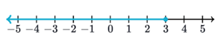Mathematics
Easy

Question

# Identify the inequality that matches the picture.## x ≠ 3    x ≤ 3    x ≥ 3    x < 3Hint:

## The correct answer is: x ≤ 3

### The blue line on the graph represents the inequality. It starts at 3 and continues backwards.Let x denotes the values satisfying the inequality.So, the variables satisfying the inequality are less than 3.Now, we have to check if the values also included 3. From the graph, at point 3 there is a closed circle. So, 3 satisfies the inequality. It’s included in the given values.The variable x is less than or equal to 3Mathematical form: x ≤ 3

We have to be careful about the limits of the inequality to solve the question.

### Related Questions to study#### With Turito Foundation.#### Get an Expert Advice From Turito.